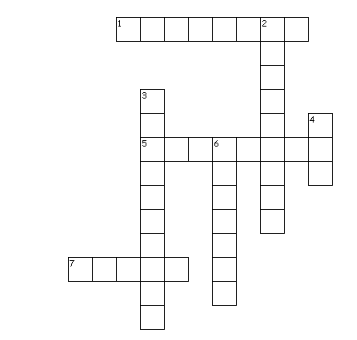# Test Paper for Linear equations

In this page we have Test Paper for Linear equations Class 8 maths . Hope you like them and do not forget to like , social share and comment at the end of the page.
Question 1
Solve each of the following equations
(a) $\frac {x+1}{4} = \frac {x-2}{2}$
(b) $1 -(p - 2) - [(p - 3) - (p - 1)] = 0$
(c) $7x - 7 = 21 -5x$
(d) $-7x = 84 +x$
(e)$0.25 (4m - 5) = 0.75m + 8$
(f) $3(x - 4) = 21$
(g)$\frac {3x}{2} - \frac {x}{3} = 8$
(h) $\frac {y}{2} -\frac {1}{4}(y- \frac {1}{3}) = \frac {1}{6} (y+1) + \frac {1}{12}$
(i) 3(2z - 3) = 12(2z + 4)

Question 2
The sum of three consecutive odd natural numbers is 69. Find the prime number out of these numbers

Question 3:
A positive number is 5 times another number. If 21 is added to both the numbers, then one of the new numbers become twice of another new numbers. Find the original numbers.

Question 4
The shifting of a number from one side of an equation to other is called
(a) Transposition
(b) Distributivity
(c) Commutativity
(d) Associativity

Question 5
Crossword PuzzleAcross
1. Shifting of variable or constant from one side to another side
5. A statement formed when an equal sign in placed between two expressions.
7. The value of x for 2x -4=10
Down
2. The values for which the equation LHS equals RHS
3. If a term of an expression consists of a number multiplied by one or more variables, this number is the __________________ of the term
4. highest power of the variable in linear equation
6. Branch of mathematics concerned with operation by symbolic numbers

Reference Books for class 8 Math

Given below are the links of some of the reference books for class 8 Math.

1. Mathematics Foundation Course for JEE/Olympiad : Class 8 This book can take students maths skills further. Only buy if child is interested in Olympiad/JEE foundation courses.
2. Mathematics for Class 8 by R S Aggarwal Detailed Mathematics book to clear basics and concepts. I would say it is a must have book for class 8 student.
3. Pearson Foundation Series (IIT -JEE / NEET) Physics, Chemistry, Maths & Biology for Class 8 (Main Books) | PCMB Combo : These set of books could help your child if he aims to get extra knowledge of science and maths. These would be helpful if child wants to prepare for competitive exams like JEE/NEET. Only buy if you can provide help to the child while studying.
4. Reasoning Olympiad Workbook - Class 8 :- Reasoning helps sharpen the mind of child. I would recommend students practicing reasoning even though they are not appearing for Olympiad.

You can use above books for extra knowledge and practicing different questions.

Note to our visitors :-

Thanks for visiting our website.